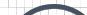Informative Information for the Uninformed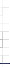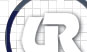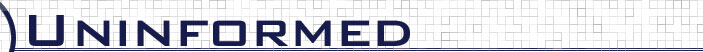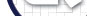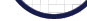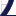Vol 1» 2005.May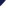# Natural Loop Detection

One of the most well known algorithms for loop detection is demonstrated in the book Compilers Principles, Techniques, and Tools by Alfred V. Aho, Ravi Sethi and Jeffrey D. Ullman. In this algorithm, the authors use a technique that consists of two components to find natural loops3.4.

The first component of natural loop detection is to build a dominator tree out of the control flow graph (CFG). A dominator can be found when all paths to a given node have to go through another node. A control flow graph is essentially a map of code execution with directional information. The algorithm in the book calls for the finding of all the dominators in a CFG. Let's look at the actual algorithm.

Starting from the entry node, the algorithm needs to check if there is a path to the slave from the entry node. This path has to avoid the master node. If it is possible to get to the slave node without touching the master node, it can be determined that the master node does not dominate the slave node. If it is not possible to get to the slave node, it is determined that the master node does dominate the slave. To implement this routine the user would call the is_path_to(ea_t from, ea_t to, ea_t avoid) function included in loop_detection.cpp. This function will essentially check to see if there is a path from the parameter from that can get to the parameter to, and will avoid the node specified in avoid. Figure 1illustrates this algorithm.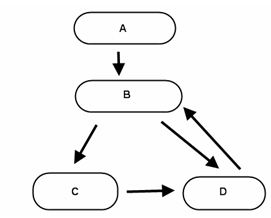As the reader can see from Figure 1, there is a loop in this CFG. Let B to C to D be the path of nodes that create a loop, it will be represented as B->C->D. There is also another loop from nodes B->D. Using the algorithm described above it is possible to verify which of these nodes is involved in the natural loop. The first question to ask is if the flow of the program can get from A to D while avoiding B. As the reader can see, it is impossible in this case to get to D avoiding B. As such, a call to the is_path_to function will tell the user that B Dominates D. This can be represented as B Dom D, and B Dom C. This is due to the fact that there is no way to reach C or D without going through B. One question that might be asked is how exactly does this demonstrate a loop? The answer is that, in fact, it doesn't. The second component of the natural loop detection checks to see if there is a link, or backedge, from D to B that would allow the flow of the program to return to node B to complete the loop. In the case of B->D there exists a backedge that does complete the loop.